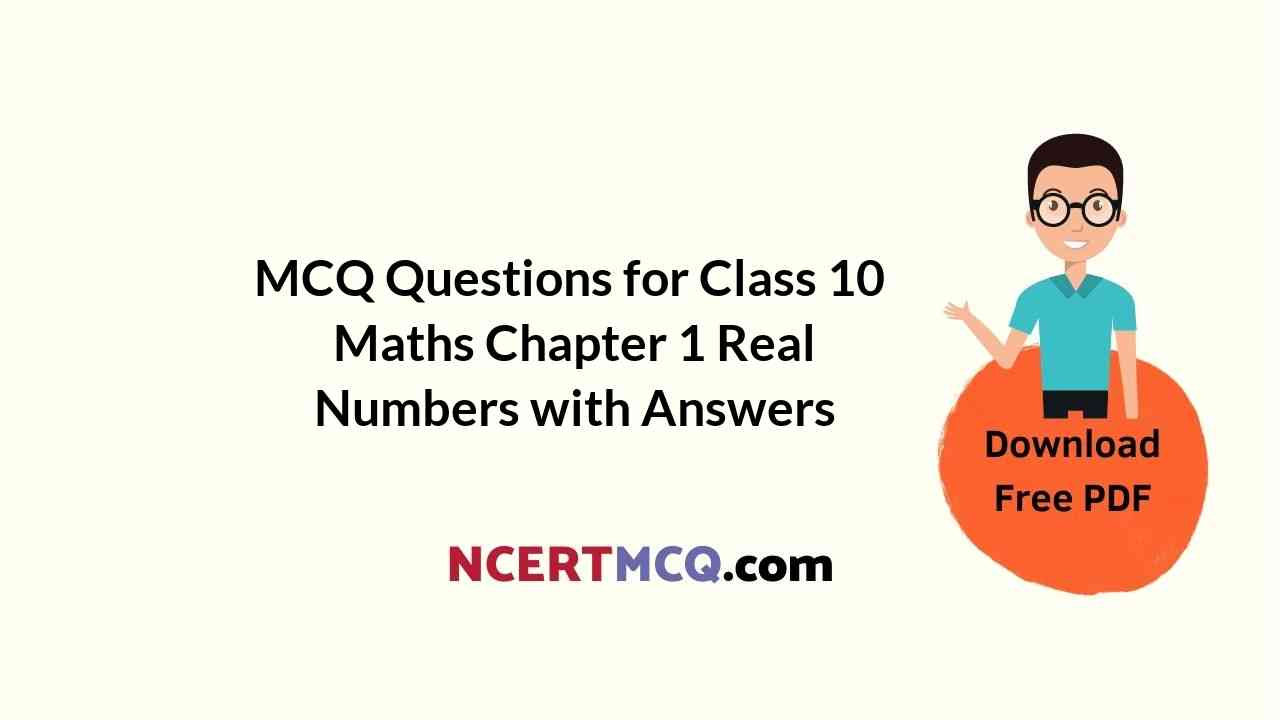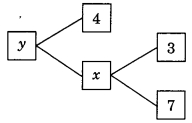Check the below NCERT MCQ Questions for Class 10 Maths Chapter 1 Real Numbers with Answers Pdf free download. MCQ Questions for Class 10 Maths with Answers were prepared based on the latest exam pattern. We have provided Real Numbers Class 10 Maths MCQs Questions with Answers to help students understand the concept very well. https://ncertmcq.com/mcq-questions-for-class-10-maths-with-answers/

Students can also refer to NCERT Solutions for Class 10 Maths Chapter 1 Real Numbers for better exam preparation and score more marks.

Real Numbers Class 10 MCQs Questions with Answers

Real Numbers Class 10 MCQ Question 1.
For any positive integer a and b, there exist unique integers q and r such that a = 3q + r, where r must satisfy.
(a) 0 ≤ r < 3
(b) 1 < r < 3
(c) 0 < r < 3
(d) 0 < r ≤ 3

Answer: (a) 0 ≤ r < 3

Class 10 Maths Chapter 1 MCQ Question 2.
The values of x and y is the given figure are(a) x + 10, y = 14
(b) x = 21, y = 84
(c) x = 21, y = 25
(d) x = 10, y = 40

Answer: (b) x = 21, y = 84

Real Numbers MCQ Class 10 Question 3.
If HCF (a, b) = 12 and a × b = 1800 then LCM (a, b) is
(a) 3600
(b) 900
(c) 150
(d) 90

Class 10 Real Numbers MCQ Question 4.
If mn = 32, where m and n are positive integers, then the value of (n)mn is
(a) 9765625
(b) 9775625
(c) 9785625
(d) 9865625

MCQ On Real Numbers Class 10 Question 5.
If ($$\frac{9}{7}$$)3 × ($$\frac{49}{81}$$)2x-6 = ($$\frac{7}{9}$$)9 then value of x is
(a) 12
(b) 9
(c) 8
(d) 6

Class 10 Maths Ch 1 MCQ Question 6.
The decimal expansion of $$\frac{17}{8}$$ will terminate after how many places of decimals?
(a) 1
(b) 2
(c) 3
(d) will not terminate

MCQ Of Real Numbers Class 10 Question 7.
The decimal expansion of n is
(a) terminating
(b) non-terminating and non-recurring
(c) non-terminating and recurring
(d) does not exist.

Chapter 1 Maths Class 10 MCQ Question 8.
If HCF of 55 and 99 is expressible in the form 55 m – 99, then the value of m:
(a) 4
(b) 2
(c) 1
(d) 3

MCQ Questions For Class 10 Maths Chapter 1 Question 9.
Given that LCM of (91, 26) = 182 then HCF (91, 26) is
(a) 13
(b) 26
(c) 7
(d) 9

Ch 1 Maths Class 10 MCQ Question 10.
The decimal expansion of number $$\frac{441}{2^2×5^3×7}$$ is
(a) A terminating decimal
(b) Non-terminating but repeating
(c) Non-terminate non repeating
(d) terminating after two places of decimal

Class 10 Maths Ch 1 MCQ Questions Question 11.
If A = 2n + 13, B = n + 7 where n is a natural number then HCF of A and B
(a) 2
(b) 1
(c) 3
(d) 4

Class 10 Maths Real Numbers MCQ Question 12.
(-1)n + (-1)8n = 0 when n is
(a) any positive integer
(b) any odd natural number
(c) any even numeral number
(d) any negative integer

Answer: (b) any odd natural number

Ch 1 Maths Class 10 MCQ Questions Question 13.
If the LCM of 12 and 42 is 10 m + 4 then the value of m is
(a) 50
(b) 8
(c) $$\frac{1}{5}$$
(d) l

Class 10 Math Chapter 1 MCQ Question 14.
The decimal expansion of the rational number $$\frac{6243}{2^2×5^4}$$ will terminate after
(a) 4 places of decimal
(b) 3 places of decimal
(c) 2 places of decimal
(d) 1 place of decimal

Answer: (a) 4 places of decimal

MCQ Class 10 Maths Chapter 1 Question 15.
n² – 1 is divisible by 8, if n is
(a) an integer
(b) a natural number
(c) an odd natural number
(d) an even natural number

Answer: (c) an odd natural number

Question 16.
If n is a natural number, then exactly one of numbers n, n + 2 and n + 1 must be a multiple of
(a) 2
(b) 3
(c) 5
(d) 7

Question 17.
The rational number between 72 and 73 is
(a) $$\frac{6}{5}$$
(b) $$\frac{3}{4}$$
(c) $$\frac{3}{2}$$
(d) $$\frac{4}{5}$$

Answer: (c) $$\frac{3}{2}$$

Question 18.
If a and 6 are two positive numbers and H and L are their HCF and LCM respectively. Then
(a) a × b = H × L
(b) a = b × H
(c) a = $$\frac{b×L}{H}$$
(d) H = $$\frac{L}{a×b}$$

Answer: (a) a × b = H × L

Question 19.
LCM of 2³ × 3² and 2² × 3³ is
(a) 2³
(b) 3³
(c) 2³ × 3³
(d) 2² × 3²

Question 20.
The LCM of 2.5, 0.5 and 0.175 is
(a) 2.5
(b) 5
(c) 7.5
(d) 0.875